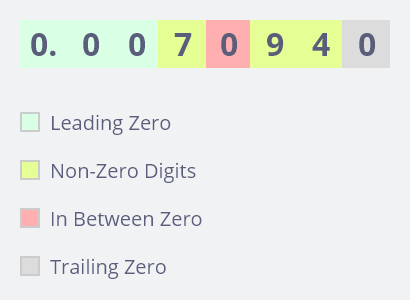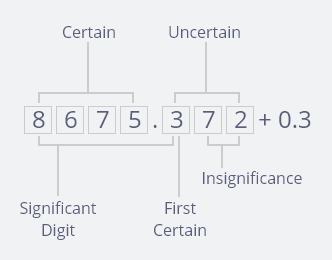Got Feedback?

Found a bug? Have a suggestion? Fill the form below and we'll take a look!

X

# Sig Fig Calculator

Significant calculator is free online tool for calculating significant figure required value, it makes our finding quick. It can convert any digit into an assigned significant figure within few seconds. This calculator performs operations on significant.
Significant calculator operators: We can share following few operators and functions, whenever we decide to find out significant figure exact value.
2. Subtraction (-)
3. Division (/)
4. Multiplication (* or x)
5. Exponent (^)
6. Parentheses
7. Functions (log and ln)

In general significant figures are used to define the degree of accuracy. A number is more accurate when it is given to greater number of significant figures.

To Determine the Number of Significant Figures, We Should Keep In Mind Three Basic Points (For prompt response)
1- Non-zero digit are always significant.
2- Any zero between two significant digits are significant.
3- A final zero or trailing zero is the decimal portion, only are significant.

## Five Rules to Identify Digits Which are Significant

Rule# 1 All non-zero digits are significant. For example, the number 8925 has 4 significant figures.

Rule# 2 All zeros between non-zero digits are significant. For example, the number 39074 has 5 significant figures.

Rule# 3 In a decimal, all zeros after non-zero digits are significant. For example, the number 4.200 has 4 significant figures.

Rule# 4 In a decimal, all zeros before a non-zero digits are not significant. For example, the number 0.00173 has 3 significant figures.

Rule# 5 In whole numbers the zeros at the end may or may not be significant. It depends on how the numbers are approximated.

## Significant Figure (A Quick Review)## Sig Fig Practice

1- 369 (3.s.f.)
2- 509 (3.s.f.)
3- 5007 (4.s.f.)
4- 500 (1.s.f.)
5- 300. (3.s.f.)
6- 300.0 (4.s.f.)
7- 0.089 (2.s.f.)
8- 0.00813 (3.s.f.)
9- 0.0089720 (5.s.f.)

## Learning objective:

Apply knowledge of significant figure in scientific calculations, any number in scientific notation are considered significant for example $$4.300 × 10^{-4}$$ has 4 significant figure present.

The significance of trailing zeros in a number not containing a decimal point can be ambiguous. For example, it may not always be clear if a number like 1300 is precise to the nearest unit (and just happens to coincidently to be an exact multiple of 100) or if it is only shown to the nearest hundred due to rounding or uncertainty various conventions exist to address this issue.
1- A bar may be placed over the last digit or the significant figure showing that any trailing zeros following this one insignificant. For example, 1300 with a bar placed over the first zero would have 3 significant figure (with the bar indicating that the number is precise to the nearest 10). i.e. 1300
2- The last significant figure of a number may be underline: For example,”2000” has two significant figures.
3- A decimal point may be placed after the number. For example, “300.” indicates specifically that three significant figures are here.

## Dealing with significant figures (A Quick Review)

1- When converting from decimal form to scientific notation, always maintain the same number of significant figures. For example 0.00017 has 2 significant figures; therefore the correct scientific notation for this number would be $$1.7 × 10^{-4}$$.
2- When multiplying and dividing numbers, the number of significant figure used to determine the original number with the smallest amount of significant figure.
3- When adding and subtracting, the final number should be rounded to the decimal point of the least precise number. For example $$1.423 × 4.2 = 6.0$$, since 1.423 has 4 significant figure and 4.2 only has 2 significant figures, the final answer must also have 2 significant figures.
4- Let’s take an example for subtraction $$234.67 – 43.5 = 191.2$$ since 43.5 has one decimal place and 234.67 has two decimal places, the final answers must have just one decimal place.
5- Let’s take another number 0.000790 start from the left side of the number don’t start counting significant figure until the first non zero number (7), then count all the way to the end of the number therefore there are three significant figure in this number i.e. (7,9,0).
6- If a number has no decimal (the decimal is absent), for example 3400 starts from the right side of the number and start counting significant figure at the first non zero number i.e. (3) so there are two significant number i.e. (3,4).
7- A zero used to fix a decimal point is never significant. The quantities 0.375, 0.0375 and 0.00375 all contain 3 significant figures. In this case, you need to think in terms of exponential numbers. 0.0375 is $$3.75 × 10^{-2}$$ (only 3 significant figures) and 0.00375 is $$3.75 × 10^{-3}$$ (again 3 significant figures). Thus 980,000 has only two significant figures. However 980,000 with the line drawn above the decimal point indicate that the measurement has 6 significant figures. 0.000000009 has only one significant figure; the remaining zeros were used to fix the decimal point.

“All digits known with certainty including the uncertain one in a measurement are called significant figure”.

## Examples

Values Significant Figures
1.479 4 S.F.
2008.9 5 S.F.
1.04000 6 S.F.
0.0000904 3 S.F.
420000 2 – 6 S.F.

In the last example it is not clear how many significant figure there are, if there are two significant figure the number represent $$420000 + -10000$$. To avoid ambiguity let’s do manipulation such numbers should always be written in exponential notation $$4.20 × 10^5$$ (3 S.F.). Very large and very small numbers are usually expressed in exponential notation. For example, $$0.0000000241 = 2.410 × 10^{-8}$$ (4 S.F). LET’S TAKE ANOTHER NUMBER, $$4000000000 = 4.0 × 108$$ (2 S.F).

Note: Only those digits before the exponent that are used to express the number of significant figures. Avoid adding the exponential term to the number of significant figure.

## How do you round off S.Fs.

1- Consider the first non-zero digit if rounding to one significant figure.
2- Consider the digit after the first non-zero digit if rounding to two significant figures.
3- Draw a vertical line after the place value digit that is required.
4- Consider the next digit.

## Rounding to S.Fs

Let’s take a number, 8263.6995

 1 S.F. 8000 8300 8260 8264

Let’s take another example, 0.49063

 1 S.F. 0.5 0.49 0.491 0.4906

Note: Trailing zeros has two conditions:
1- Trailing zeros in a number without decimal is not considered as a significant. For example 3490000 has (3 S.F). 2935000 has (4 S.F).
2- The number with decimal: Trailing zeros in a number with decimal is considered significant. For example 17.800 has (5 S.F), 26.3900 has (6 S.F).

## FAQS

Q1: What is the importance of significant figure?

Answer: Significant figures are an important part of scientific and mathematical calculation. There are three basic aspects;
1- Accuracy
2- Precision
3- Uncertainty

Q2: What is meant by accuracy in sig fig?

Answer: Accuracy means how close the reported value is to the actual value.

Q3: What is meant by precision?

Answer: Precision is a measure of how small the smallest reportable difference is.

Q4: What is meant by uncertainty in sig fig?

Answer: Uncertainty is just an estimate and thus it cannot be more precise then the best estimate of measured value.

Q5: What is the pictorial representation of a sig fig?NOTE: All of the digits in a measurement including the uncertain last digit are known significant figures/digits.

Q6: What is the definition of significant figure?

Answer: The significant figure actually refers to particular digit in a number those are sometimes called significant digit.

Q7: Why is it important to calculate significant figure?

Answer: Significant figure are integral part of scientific and mathematical calculation as it deal with the precision and accuracy of figure.

Q8: Why do we need a significant figure?

Answer: We need sig fig for more precision and accuracy as it play the magical role in calculating the final result of the end value.

Q9: How do you explain significant figure?

Answer: The number of significant figure in a result is simply the number of figures that are known with some degree of accuracy or reliability the number 19.4 is said to have three significant figure the number 19.40 is said to have four significant figure.

Q10: How do you calculate significant figure?

Answer: To find out the number of significant figure in a specific number consider the basic three rules:
1- Non zero digits are always significant
2- Any zeros between two significant digits are significant
3- A final zero or trailing zero in the decimal portion only are significant.

Q11: How do you round significant figures?

1- Look at the first non zero digits if rounding to one significant digit.
2- Look at the digit after the very first non zero digit if rounding to two significant.
3- Draw a vertical line after the place value digit that is required.
4- Consider the next digit.

Q12: What is a 4 digit significant figure?

Answer: The fourth significant figure of a number is the digit after the third significant number.

Q13: How many sig figs in 1000?

Answer: Thousand has one significant figure, as trailing zeros do not consider in significant figure, if there is no decimal point.

Q14: How many significant figures are in the number 0.01030?

Answer: There are only 5 S.F.

Q15: Which of the following has the smallest number of significant figures, 0.000401, 223 and 2.05?

Q16: How many significant figures are in the number 70.010?

Q17: How many significant figures are in number 0.01000?

Answer: This is tricky and requires prior knowledge. As an exponential number it can be represented as $$1.000 × 10^{-2}$$ , now it is clear that there are 4 S.F.

Q18: How many significant figures in 100?

Answer: 100 has one significant figure, as trailing zeros do not consider in significant figure, if there is no decimal point.

• Embed Calculator Widget
• Direct URL